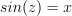/NumPy 1.17

# numpy.arcsin

`numpy.arcsin(x, /, out=None, *, where=True, casting='same_kind', order='K', dtype=None, subok=True[, signature, extobj]) = <ufunc 'arcsin'>`

Inverse sine, element-wise.

Parameters: `x : array_like` `y`-coordinate on the unit circle. `out : ndarray, None, or tuple of ndarray and None, optional` A location into which the result is stored. If provided, it must have a shape that the inputs broadcast to. If not provided or `None`, a freshly-allocated array is returned. A tuple (possible only as a keyword argument) must have length equal to the number of outputs. `where : array_like, optional` This condition is broadcast over the input. At locations where the condition is True, the `out` array will be set to the ufunc result. Elsewhere, the `out` array will retain its original value. Note that if an uninitialized `out` array is created via the default `out=None`, locations within it where the condition is False will remain uninitialized. **kwargs For other keyword-only arguments, see the ufunc docs. `angle : ndarray` The inverse sine of each element in `x`, in radians and in the closed interval `[-pi/2, pi/2]`. This is a scalar if `x` is a scalar.

#### Notes

`arcsin` is a multivalued function: for each `x` there are infinitely many numbers `z` such that. The convention is to return the angle `z` whose real part lies in [-pi/2, pi/2].

For real-valued input data types, arcsin always returns real output. For each value that cannot be expressed as a real number or infinity, it yields `nan` and sets the `invalid` floating point error flag.

For complex-valued input, `arcsin` is a complex analytic function that has, by convention, the branch cuts [-inf, -1] and [1, inf] and is continuous from above on the former and from below on the latter.

The inverse sine is also known as `asin` or sin^{-1}.

#### References

Abramowitz, M. and Stegun, I. A., Handbook of Mathematical Functions, 10th printing, New York: Dover, 1964, pp. 79ff. http://www.math.sfu.ca/~cbm/aands/

#### Examples

```>>> np.arcsin(1)     # pi/2
1.5707963267948966
>>> np.arcsin(-1)    # -pi/2
-1.5707963267948966
>>> np.arcsin(0)
0.0
```

© 2005–2019 NumPy Developers
Licensed under the 3-clause BSD License.
https://docs.scipy.org/doc/numpy-1.17.0/reference/generated/numpy.arcsin.html